Question

# Organ pipe A with both ends open has a fundamental frequency of 320.0 Hz. The third...

Organ pipe A with both ends open has a fundamental frequency of 320.0 Hz. The third harmonic of organ pipe B with one end open has the same frequency as the second harmonic of pipe A. Assume a speed of sound of 343 m/s.
What is the length of Pipe A? What is the length of Pipe B?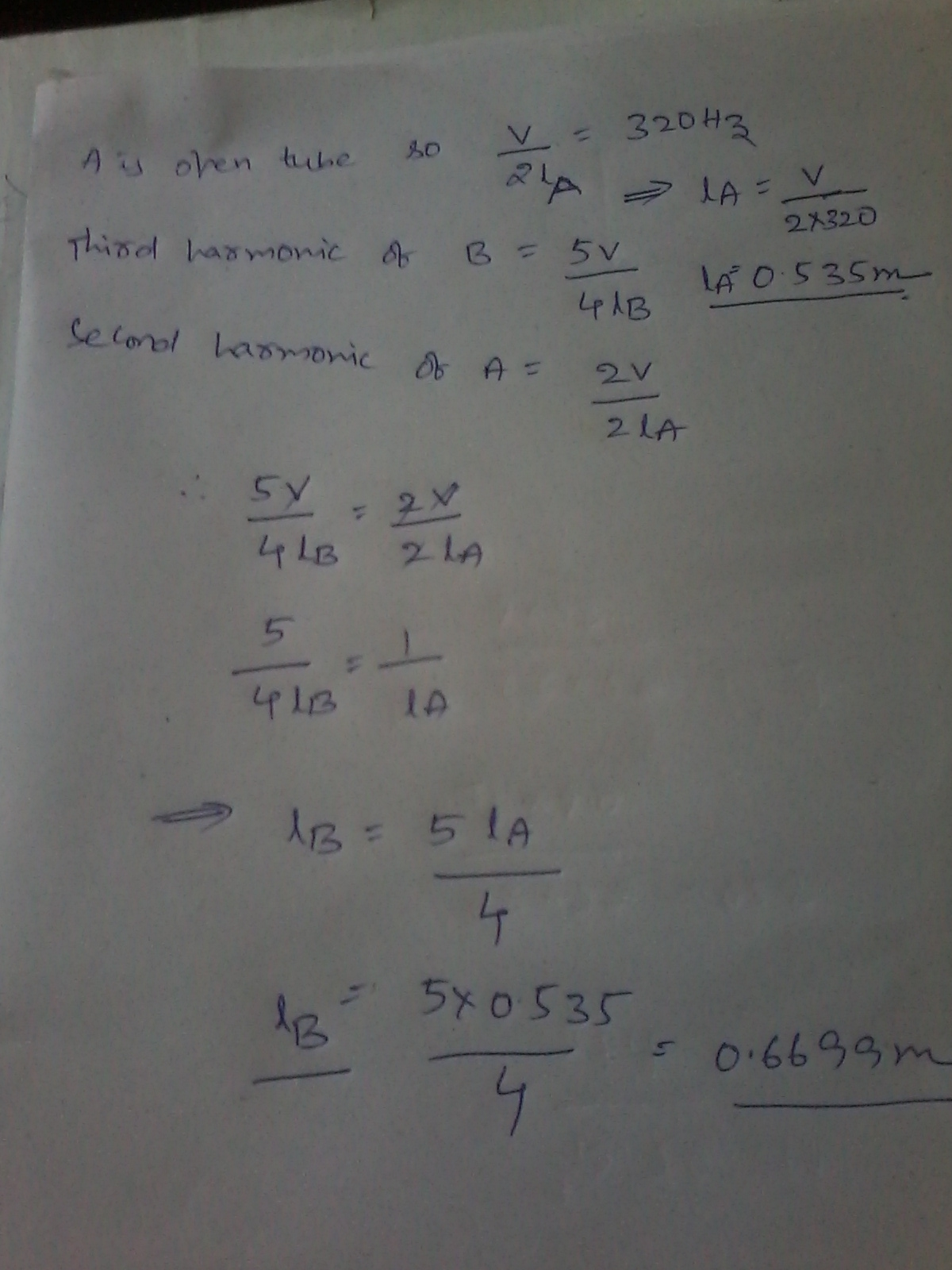#### Earn Coins

Coins can be redeemed for fabulous gifts.

Similar Homework Help Questions
• ### Question 13: A tube with both ends open has fundamental frequency of 300 Hz. The second...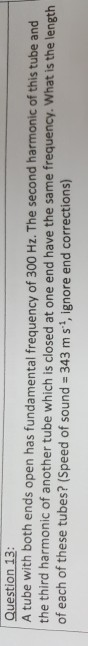Question 13: A tube with both ends open has fundamental frequency of 300 Hz. The second harmonic of this tube and the third harmonic of another tube which is closed at one end have the same frequency. What is the length of each of these tubes? (Speed of sound 343 m s, ignore end corrections)

• ### Calculate the length of a pipe that has a fundamental frequency of 316 Hz. (Take the...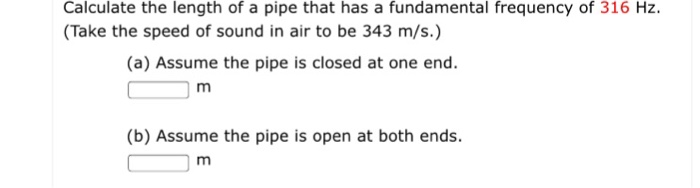Calculate the length of a pipe that has a fundamental frequency of 316 Hz. (Take the speed of sound in air to be 343 m/s.) Calculate the length of a pipe that has a fundamental frequency of 316 Hz. (Take the speed of sound in air to be 343 m/s.) (a) Assume the pipe is closed at one end (b) Assume the pipe is open at both ends

• ### church organ pipe is open at both ends. The third (m 3) harmonic has frequency 262...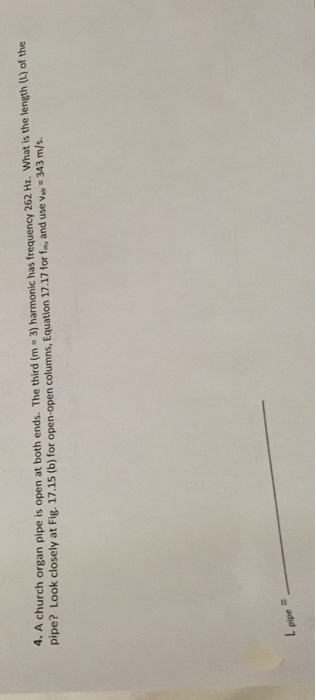church organ pipe is open at both ends. The third (m 3) harmonic has frequency 262 Hz. What is the length (L) of the pipe? Look closely at Fig. 17.15 (b) for open-open columns, Equation 17.17 for fm, and use var 343 m/s. L pipe

• ### Calculate the length of a pipe that has a fundamental frequency of 997 Hz. (Take the...

Calculate the length of a pipe that has a fundamental frequency of 997 Hz. (Take the speed of sound in air to be 343 m/s.) (a) Assume the pipe is closed at one end. m (b) Assume the pipe is open at both ends. m

• ### The fundamental of an organ pipe that is closed at one end and open at the...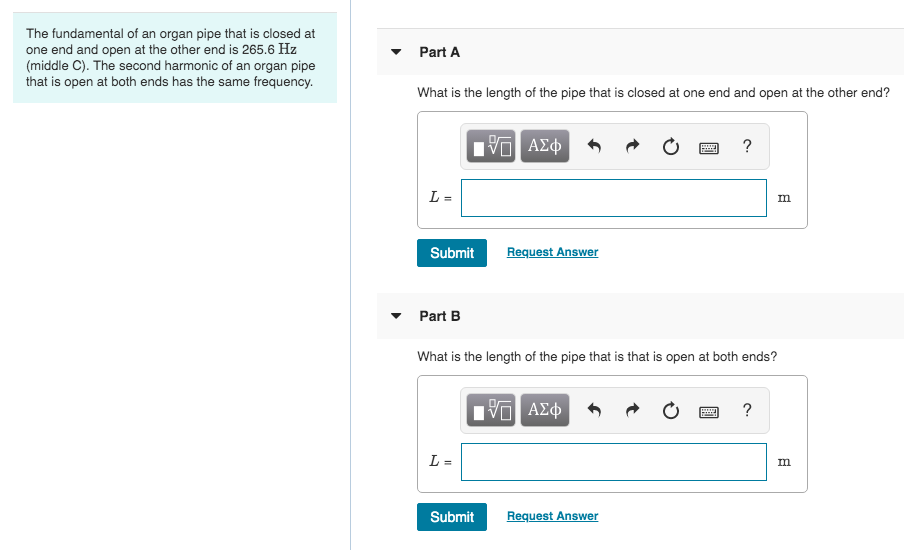The fundamental of an organ pipe that is closed at one end and open at the other end is 265.6 Hz (middle C). The second harmonic of an organ pipe that is open at both ends has the same frequency. Part A What is the length of the pipe that is closed at one end and open at the other end? PO AJĄ O O ? Submit Request Answer Part B What is the length of the pipe that is...

• ### 4. An organ pipe open at both ends has the second harmonic of 512 Hz. What...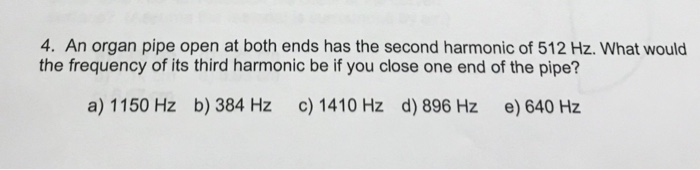4. An organ pipe open at both ends has the second harmonic of 512 Hz. What would the frequency of its third harmonic be if you close one end of the pipe? a) 1150 Hz b) 384 Hz c) 1410 Hz d) 896 Hz e) 640 Hz

• ### A certain organ pipe, open at both ends, produces a fundamental frequency of 290 Hz in...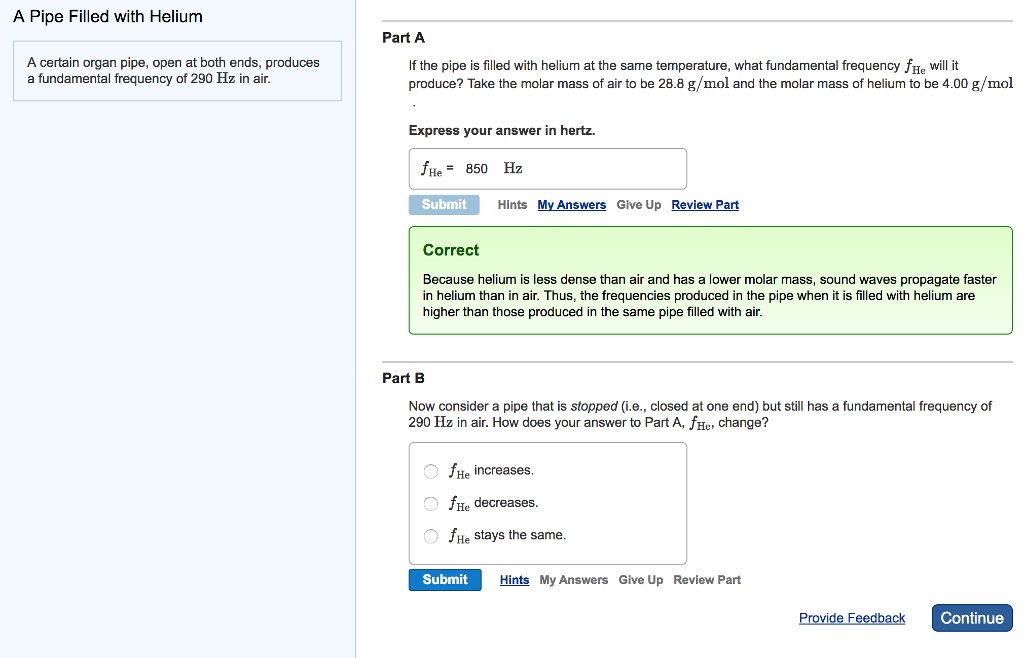A certain organ pipe, open at both ends, produces a fundamental frequency of 290 Hz in air. If the pipe is filled with helium at the same temperature, what fundamental frequency f_He will it produce? Take the molar mass of air to be 28.8 g/mol and the molar mass of helium to be 4.00 g/mol Express your answer in hertz. Now consider a pipe that is stopped (i.e., closed at one end) but still has a fundamental frequency of 290...

• ### 2B.5 An organ pipe open at both ends has a fundamental frequency of 440 Hz (concert...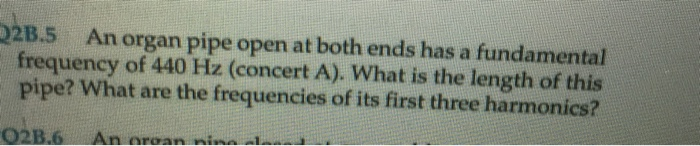2B.5 An organ pipe open at both ends has a fundamental frequency of 440 Hz (concert A). What is the length of this pipe? What are the frequencies of its first three harmonics? 02B.6 An aroan nina

• ### (8) Calculate the length of a pipe that has a fundamental frequency of 1085 Hz, assuming...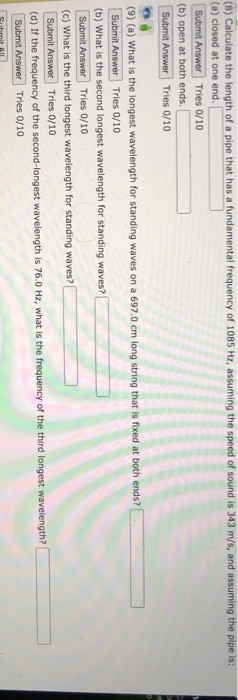(8) Calculate the length of a pipe that has a fundamental frequency of 1085 Hz, assuming the speed of sound is 343 m/s, and assuming the pipe is: (a) closed at one end. Submit Answer Tries 0/10 (b) open at both ends. Submit Answer Tries 0/10 (9) (a) What is the longest wavelength for standing waves on a 697.0 cm long string that is fixed at both ends? Submit Answer Tries 0/10 (b) What is the second longest wavelength for...

• ### Pipe A, which is 2.40 m long and open at both ends, oscillates at its third...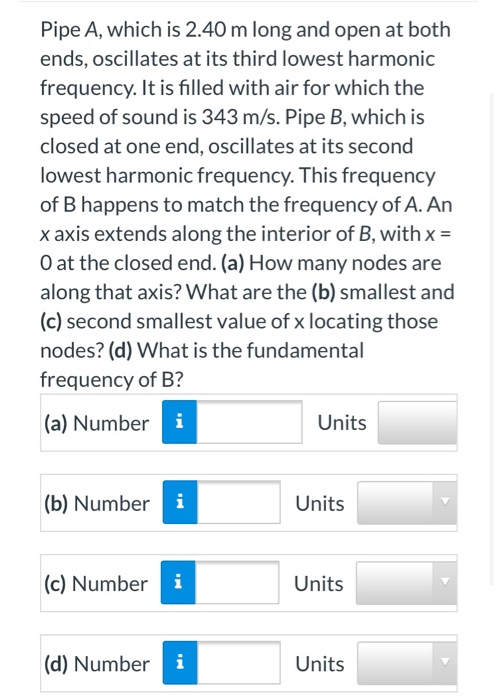Pipe A, which is 2.40 m long and open at both ends, oscillates at its third lowest harmonic frequency. It is filled with air for which the speed of sound is 343 m/s. Pipe B, which is closed at one end, oscillates at its second lowest harmonic frequency. This frequency of B happens to match the frequency of A. An x axis extends along the interior of B, with x = O at the closed end. (a) How many nodes...